# SUMIF in Google Sheets with formula examples

The tutorial shows how to use the SUMIF function in Google spreadsheets to conditionally sum cells. You will find formula examples for text, numbers and dates and learn how to sum with multiple criteria.

Some of the best functions in Google Sheets are those that help you summarize and categorize data. Today, we are going to have a closer look at one of such functions - SUMIF - a powerful instrument to conditionally sum cells. Before studying the syntax and formula examples, let me begin with a couple of important remarks.

Google Sheets has two functions to add up numbers based on conditions: SUMIF and SUMIFS. The former evaluates just one condition while the latter can test multiple conditions at a time. In this tutorial, we will focus solely on the SUMIF function, the use of SUMIFS will be covered in the next article.

If you know how to use SUMIF in Excel desktop or Excel online, SUMIF in Google Sheets will be a piece of cake for you since both are essentially the same. But don't rush to close this page yet - you may find a few unobvious but very useful SUMIF formulas you didn't know!

## SUMIF in Google Sheets - syntax and basic uses

The SUMIF function is Google Sheets is designed to sum numeric data based on one condition. Its syntax is as follows:

SUMIF(range, criterion, [sum_range])

Where:

• Range (required) - the range of cells that should be evaluated by criterion.
• Criterion (required) - the condition to be met.
• Sum_range (optional) - the range in which to sum numbers. If omitted, then range is summed.

As an example, let's make a simple formula that will sum numbers in column B if column A contains an item equal to the "sample item".

For this, we define the following arguments:

• Range - a list of items - A5:A13.
• Criterion - a cell containing the item of interest - B1.
• Sum_range - amounts to be summed - B5:B13.

Putting all the arguments together, we get the following formula:

`=SUMIF(A5:A13,B1,B5:B13)`

And it works exactly as it should: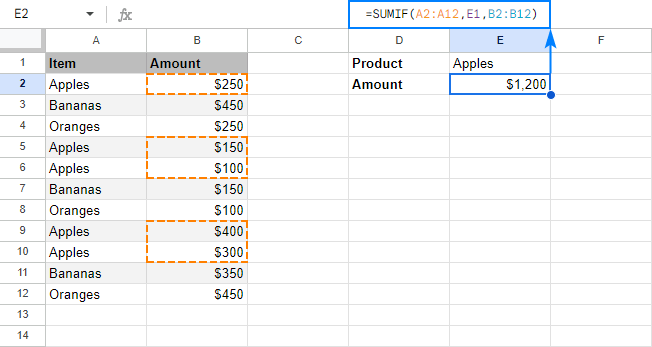From the above example, you may have the impression that using SUMIF formulas in Google spreadsheets is so easy that you could do it with your eyes shut. In most cases, it is really so :) But still there are some tricks and non-trivial uses that could make your formulas more effective. The below examples demonstrate a few typical use cases. To make the examples easier to follow, I invite you to open our sample SUMIF Google Sheet.

### SUMIF formulas with text criteria (exact match)

To add up numbers that have a specific text in another column in the same row, your simply supply the text of interest in the criterion argument of your SUMIF formula. As usual, any text in any argument of any formula should be enclosed in "double quotes".

For example, to get a total of bananas, you use this formula:

`=SUMIF(A5:A13,"bananas",B5:B13)`

Or, you can put the criterion in some cell and refer to that cell:

`=SUMIF(A5:A13,B1,B5:B13)`

This formula is crystal clear, isn't it? Now, how do you get a total of all items except bananas? For this, use the not equal to operator:

`=SUMIF(A5:A13,"<>bananas",B5:B13)`

If an "exclusion item" is input in a cell, then you enclose the not equal to operator in double quotes ("<>") and concatenate the operator and cell reference by using an ampersand (&). For example:

`=SUMIF (A5:A13,"<>"&B1, B5:B13)`

The following screenshot demonstrates both "Sum if equal to" and "Sum if not equal to" formulas in action: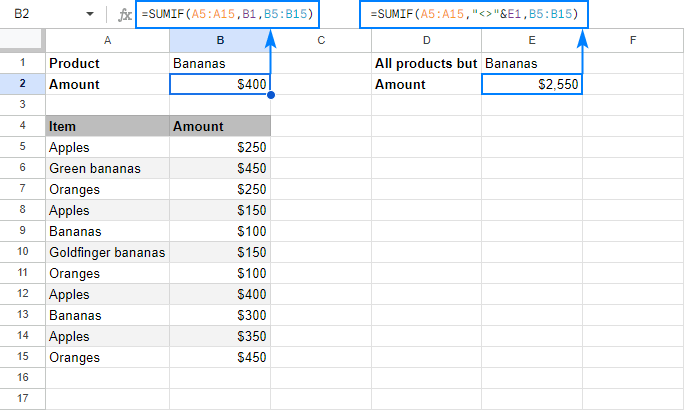Please note that SUMIF in Google Sheets searches for the specified text exactly. In this example, only Bananas amounts are summed, Green bananas and Goldfinger bananas are not included. To sum with partial match, use wildcard characters as shown in the next example.

### SUMIF formulas with wildcard characters (partial match)

In situations when you want to sum cells in one column if a cell in another column contains a specific text or character as part of the cell contents, include one of the following wildcards in your criteria:

• Question mark (?) to match any single character.
• Asterisk (*) to match any sequence of characters.

For example, to sum the amounts of all sorts of bananas, use this formula:

`=SUMIF(A5:A13,"*bananas*",B5:B13)`

You can also use wildcards together with cell references. For this, enclose the wildcard character in quotation marks, and concatenate it with a cell reference:

`=SUMIF(A5:A13, "*"&B1&"*", B5:B13)`

Either way, our SUMIF formula adds up the amounts of all bananas: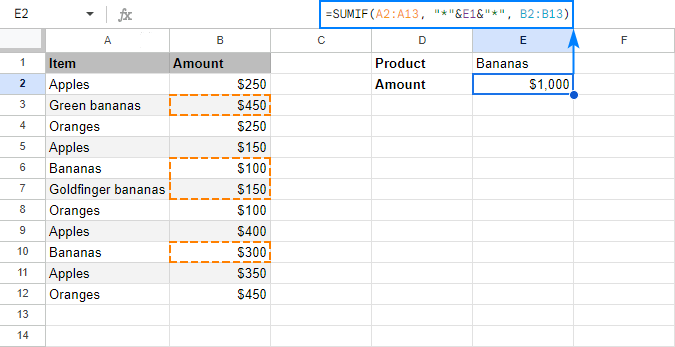To match an actual question mark or asterisk, prefix it with the tilde (~) character like "~?" or "~*".

For example, to sum numbers in column B that have an asterisk in column A in the same row, use this formula:

`=SUMIF(A5:A13, "~*", B5:B13)`

You can even type an asterisk in some cell, say B1, and concatenate that cell with the tilde char:

`=SUMIF(A5:A13, "~"&B1, B5:B13)`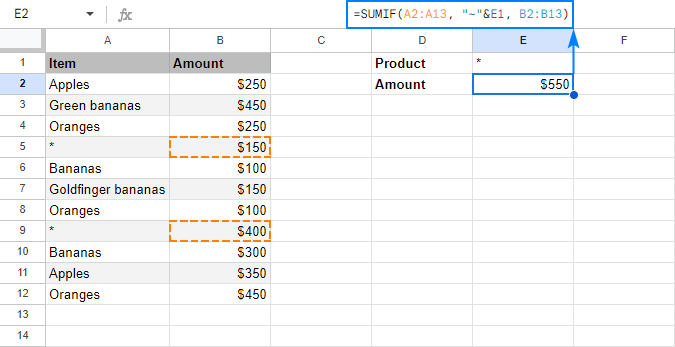### Case-sensitive SUMIF in Google Sheets

By default, SUMIF in Google Sheets does not see the difference between small and capital letters. For force it to teat uppercase and lowercase characters differently, use SUMIF in combination with the FIND and ARRAYFORMULA functions:

SUMIF(ARRAYFORMULA( FIND("text", range)), 1, sum_range)

Supposing you have a list of order numbers in A5:A13 and corresponding amounts in C5:C13, where the same order number appears in several rows. You enter the target order id in some cell, say B1, and use the following formula to return the order total:

`=SUMIF(ARRAYFORMULA(FIND(B1, A5:A13)),1, C5:C13)`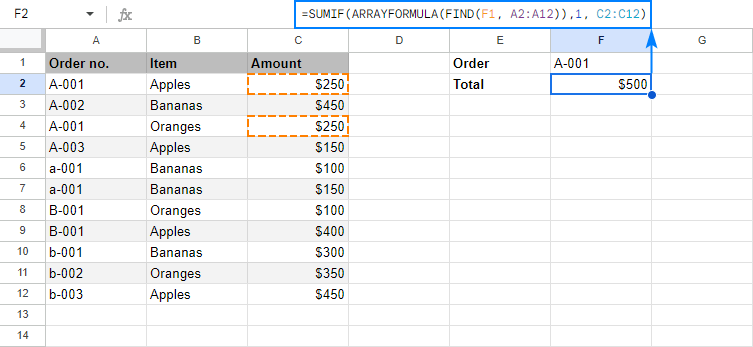#### How this formula works

To better understand the formula's logic, let's break it down into the meaningful parts:

The trickiest part is the range argument: ARRAYFORMULA(FIND(B1, A5:A13))

You use the case-sensitive FIND function to look for the exact order id. The problem is that a regular FIND formula can only search within a single cell. To search within a range, an array formula is needed, so you nest FIND inside ARRAYFORMULA.

When the above combination finds an exact match, it returns 1 (the position of the first found character), otherwise a #VALUE error. So, the only thing left for you to do is to sum the amounts corresponding to 1's. For this, you put 1 in the criterion argument, and C5:C13 in the sum_range argument. Done!

### SUMIF formulas for numbers

To sum numbers that meet a certain condition, use one of the comparison operators in your SUMIF formula. In most cases, choosing an appropriate operator is not a problem. Embedding it in the criterion properly could be a challenge.

#### Sum if greater than or less than

To compare the source numbers to a particular number, use one of the following logical operators:

• greater than (>)
• less than (<)
• greater than or equal to (>=)
• less than or equal to (<=)

For example, to add up numbers in B5:B13 that are greater than 200, use this formula:

`=SUMIF(B5:B13, ">200")`

Please notice the correct syntax of the criterion: a number prefixed with a comparison operator, and the whole construction enclosed in quotation marks.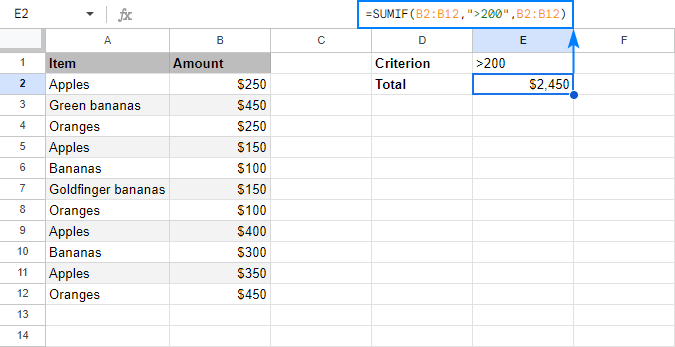Or, you can type the number in some cell, and concatenate the comparison operator with a cell reference:

`=SUMIF(B5:B13, ">"&B1, B5:B13)`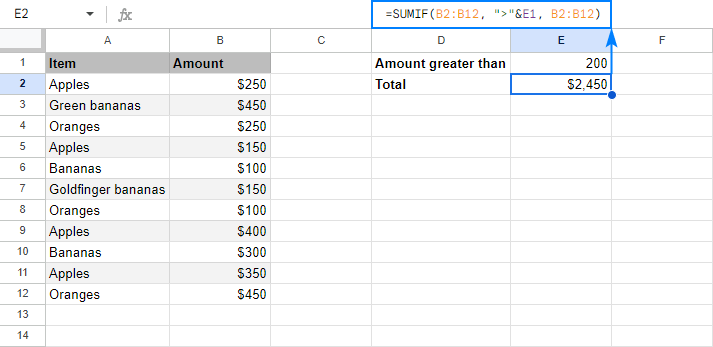You can even input both the comparison operator and number in separate cells, and concatenate those cells: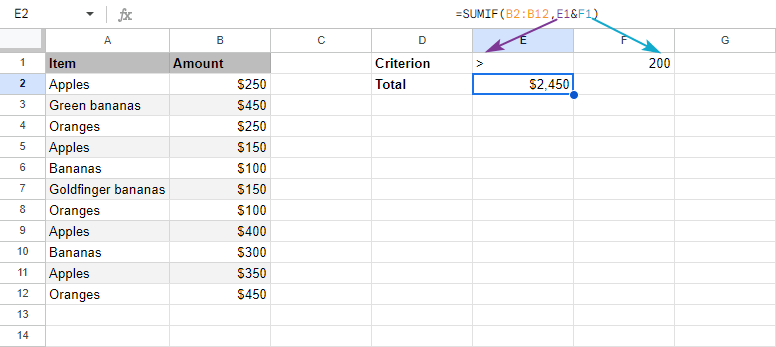In a similar manner, you can use other logical operators such as:

Sum if greater than or equal to 200:

`=SUMIF(B5:B13, ">=200")`

Sum if less than 200:

`=SUMIF(B5:B13, "<200")`

Sum if less than or equal to 200:

`=SUMIF(B5:B13, "<=200")`

#### Sum if equal to

To sum numbers that equal a specific number, you can use the equality sign (=) together with the number or omit the equality sign and include only the number in the criterion argument.

For example, to add up amounts in column B whose quantity in column C is equal to 10, use any of the below formulas:

`=SUMIF(C5:C13, 10, B5:B13)`

or

`=SUMIF(C5:C13, "=10", B5:B13)`

or

`=SUMIF(C5:C13, B1, B5:B13)`

Where B1 is the cell with the required quantity.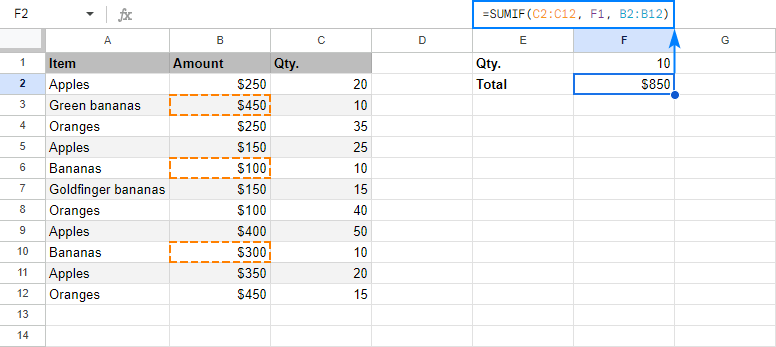#### Sum if not equal to

To sum numbers other than the specified number, use the not equal to operator (<>).

In our example, to add up the amounts in column B that have any quantity except 10 in column C, go with one of these formulas:

`=SUMIF(C5:C13, "<>10", B5:B13)`

`=SUMIF(C5:C13, "<>"&B1, B5:B13)`

The screenshot below shows the result: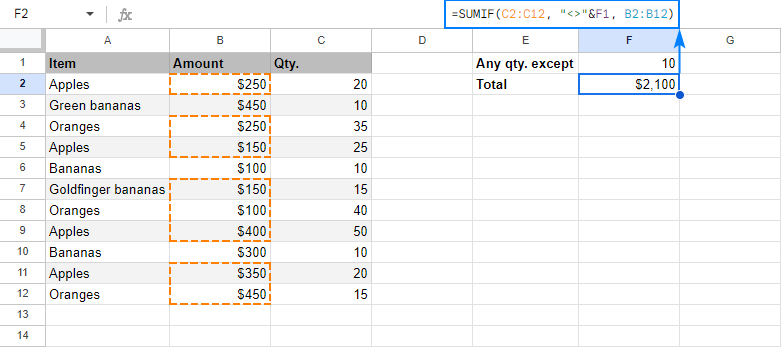### Google Sheets SUMIF formulas for dates

To conditionally sum values based on date criteria, you also use the comparison operators like shown in the above examples. The key point is that a date should be supplied in the format that Google Sheets can understand.

For instance, to sum amounts in B5:B13 for delivery dates prior to 11-Mar-2018, build the criterion in one of these ways:

`=SUMIF(C5:C13, "<3/11/2018", B5:B13)`

`=SUMIF(C5:C13, "<"&DATE(2018,3,11), B5:B13)`

`=SUMIF(C5:C13, "<"&B1, B5:B13)`

Where B1 is the target date: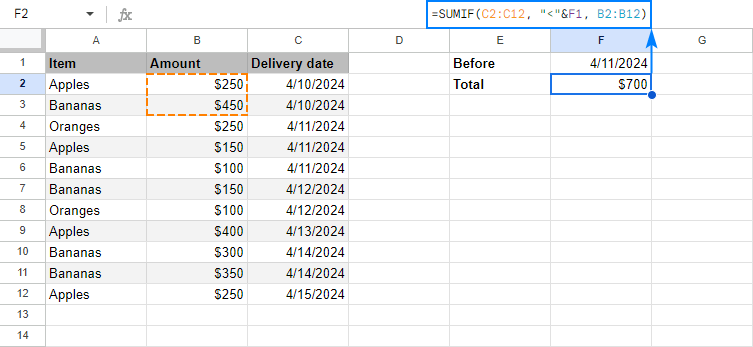In case you want to conditionally sum cells based on today's date, include the TODAY() function in the criterion argument.

As an example, let's make a formula that adds up the amounts for today's deliveries:

`=SUMIF(C5:C13, TODAY(), B5:B13)`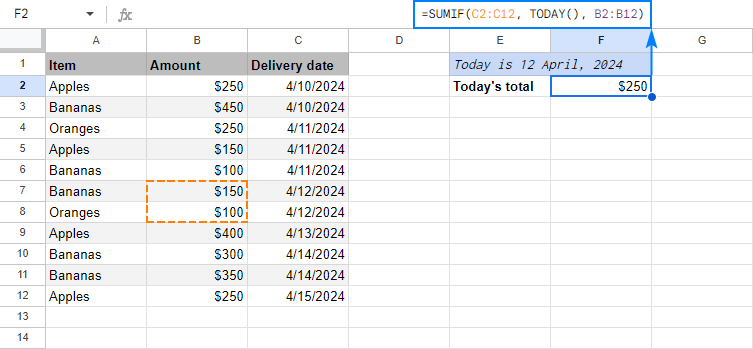Taking the example further, we can find a total of past and future deliveries:

Before today: `=SUMIF(C5:C13, "<"&TODAY(), B5:B13)`

After today: `=SUMIF(C5:C13, ">"&TODAY(), B5:B13)`

### Sum based on blank or non-blank cells

In many situations, you may need to sum values in a certain column if a corresponding cell in another column is or is not empty.

For this, use one of the following criteria in your Google Sheets SUMIF formulas:

#### Sum if blank:

• "=" to sum cells that are completely blank.
• "" to sum blank cells including those that contain zero length strings.

#### Sum if not blank:

• "<>" to add up cells that contain any value, including zero length strings.

For example, to sum the amounts for which the delivery date is set (a cell in column C is not empty), use this formula:

`=SUMIF(C5:C13, "<>", B5:B13)`

To get a total of the amounts with no delivery date (a cell in column C is empty), use this one:

`=SUMIF(C5:C13, "", B5:B13)`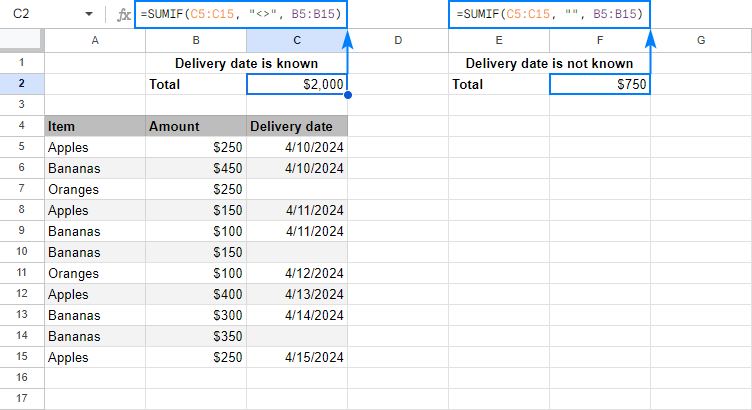### Google Sheets SUMIF with multiple criteria (OR logic)

The SUMIF function in Google Sheets is designed to add up values based on just one criterion. To sum with multiple criteria, you can add two or more SUMIF functions together.

For example, to sum Apples and Oranges amounts, utilize this formula:

`=SUMIF(A6:A14, "apples", B6:B14)+SUMIF(A6:A14, "oranges", B6:B14)`

Or, put the item names in two separate cells, say B1 and B2, and use each of those cells as a criterion:

`=SUMIF(A6:A14, B1, B6:B14)+SUMIF(A6:A14, B2, B6:B14)`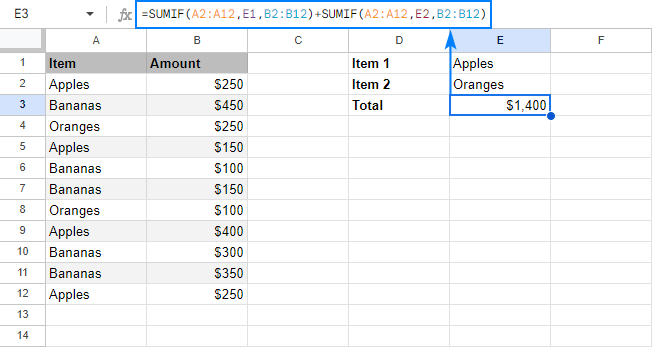Please note that this formula works like SUMIF with OR logical - it sums values if at least one of the specified criteria is met.

In this example, we add values in column B if column A equals "apples" or "oranges". In other words, SUMIF() + SUMIF() works like the following pseudo-formula (not a real one, it only demonstrates the logic!): sumif(A:A, "apples" or "oranges", B:B).

If you are looking to conditionally sum with AND logical, i.e. add up values when all of the specified criteria are met, use the Google Sheets SUMIFS function.

### Google Sheets SUMIF - things to remember

Now that you know the nuts and bolts of the SUMIF function in Google Sheets, it may be a good idea to make a short summary of what you've already learned.

#### 1. SUMIF can evaluate only one condition

The syntax of the SUMIF function allows for only one range, one criterion and one sum_range. To sum with multiple criteria, either add several SUMIF functions together (OR logic) or use SUMIFS formulas (AND logic).

#### 2. The SUMIF function is case-insensitive

If you are looking for a case-sensitive SUMIF formula that can differentiate between uppercase and lowercase characters, use SUMIF in combination with ARRAYFORMULA and FIND as shown in this example.

#### 3. Supply equally sized range and sum_range

In fact, the sum_range argument specifies only the upper leftmost cell of the range to sum, the remaining area is defined by the dimensions of the range argument.

To put it differently, SUMIF(A1:A10, "apples", B1:B10) and SUMIF(A1:A10, "apples", B1:B100) will both sum values in the range B1:B10 because it is the same size as range (A1:A10).

So, even if you mistakenly supply a wrong sum range, Google Sheets will still calculate your formula right, provided the top left cell of sum_range is correct.

That said, it is still recommended to provide equally sized range and sum_range to avoid mistakes and prevent inconsistency issues.

#### 4. Mind the syntax of SUMIF criteria

For your Google Sheets SUMIF formula to work correctly, express the criteria the right way:

• If the criterion includes text, wildcard character or logical operator followed by a number, text or date, enclose the criterion in quotation marks. For example:

`=SUMIF(A2:A10, "apples", B2:B10)`

`=SUMIF(A2:A10, "*", B2:B10)`

`=SUMIF(A2:A10, ">5")`

`=SUMIF(A5:A10, "<>apples", B5:B10)`

• If the criterion includes a logical operator and a cell reference or another function, use the quotation marks to start a text string and ampersand (&) to concatenate and finish the string off. For example:

`=SUMIF(A2:A10, ">"&B2)`

`=SUMIF(A2:A10, ">"&TODAY(), B2:B10)`

#### 5. Lock ranges with absolute cell references if needed

If you plan to copy or move your SUMIF formula at a later point, fix the ranges by using absolute cell references (with the \$ sign) like in SUMIF(\$A\$2:\$A\$10, "apples", \$B\$2:\$B\$10).

This is how you use the SUMIF function in Google Sheets. To have a closer look at the formulas discussed in this tutorial, you are welcome to open our sample SUMIF Google Sheet. I thank you for reading and hope to see you on our blog next week!

### 67 responses to "SUMIF in Google Sheets with formula examples"

1.Kathryn Mazie says:

Please notice that I would like to have more of Excel Tutorial in lesson, because what I'm during now is very helpful to me

I'm learning an lot from theses lesson that I bring up off your system. I appreciate Excel and the lesson's that are giving to me when I'm uploading theses tutorial

2.Brian says:

Very thorough tutorial. Love it! I've been trying to get a simple SUMIF to work and have it reference columns on another tab but Sheets does not seem to like this. Have you been able to get it to work?

Thanks!

ex.

Tab to be referenced in the same sheet is called "Joint Chequing".

=SUMIF('Joint Chequing'!C2:C1001, A1, 'Joint Chequing'!D2:D1001)

3.moshe rabenu says:

Thanks a lot! this is very useful

4.Stefan says:

thanks a lot for these great tips!

5.Aneesh says:

How to do reference from a different sheet itself?

•Natalia Sharashova (Ablebits.com Team) says:

Thank you for your question, Aneesh.

To reference a cell/range from another sheet, first enter the required sheet name wrapped in single quotes, then put an exclamation mark (!), and then enter the range you refer to, for example:
=SUMIF('Sheet2'!B15:B26,">20")

6.Akobir says:

Hi,

Indeed, helpful. Thank you. But i would like you to help me to use sumifs between two or more sheets. Thanks.

7.L S says:

This is useful...However, I am looking to create a sheet that has buyer numbers and the costs of what they bought in it. So I will sort by buyer number and then want to sum what they owe. How do I use the SUMIF when I don't know what the criteria is (if buyer #2 bought 3 items, I want to sum those three columns; then if buyer # 3 bought 6 items, I want it to sum those 6 columns) I don't know how many items they will have bought. I hope that makes sense.... thanks!

8.Julien says:

Hi!
I would like to know if there are some optimisations about SUMIF.
I mean, is the order of criteria important for the SUMIF? (stop the check when a criterion is false) For the very massive sheets, could be interesting.
Thank you.

9.Robin says:

I'm trying to use this formula to put a value in a cell on a tab called 'overview' that calculates the sum of entries in column c of a tab called 'visit reports' that contain the word school in column b of the 'visit reports' tab. =SUMIF ('Visit reports'!B1:B300, "*school*",C1:C300). It is returning zero even when I have values in both column b and c. The sheet is at https://docs.google.com/spreadsheets/d/1PQFS5_fkhGGfR_2TcAudT9KKE98s8oq0L8zlcES3mNo/edit?usp=sharing. Can anyone help?

•Fred says:

Wondering if you need to put the sheet name with the sum range too.
Looks like you managed to figure it out though.

•Abear Awadallah says:

Hi.. were you able to figure out how to solve this? Can't get access into the sheet..

•Natalia Sharashova (Ablebits.com Team) says:

Hi Abear,

If you describe your task for me and specify the formula you're trying to use, I'll do my best to help you out.

10.Scooter McFoo says:

Can you add a note that when testing for equality, you don't need to specify a comparison operator?

11.Jason says:

You nailed it, answered my question quickly, thanks!

12.Michael says:

Hi Svetlana,
Thank you very much for writing and sharing such an excellent guide to using SUMIF in Google Sheets. It greatly improved my ability to analyze my data. After a good bit of searching, it was the only tutorial I found that provided the knowledge I needed in a manner that not only showed me how to write the formulas but enabled me to fully understand the concepts behind the formula. Of-course I stopped looking once I found yours, but I have a hard time imagining there is a better one out there.

I really liked how you structured this tutorial. It was a perfect mix of concepts and examples. The progression from simple to more complex cases made it possible for me to understand and implement the more complex scenarios. Well done!

I'm looking forward to your tutorial on SUMIFS!

13.Yair says:

I tried using what you wrote in the "SUMIF formulas with wildcard characters (partial match)" section and I keep getting 0.

The formula works just fine with actual characters, but once I swap any of them with the "?" wildcard it always returns 0.

•Yair says:

My bad, I didn't know I have to use date(yyyy,mm,dd) if working with dates.

It does seem to be the case that wildcards don't work within that formula though.

•Natalia Sharashova (Ablebits.com Team) says:

Hello Yair,

Would you mind sharing your formula with us? Perhaps, we'll notice right away if anything is incorrect there.

14.Meg says:

Hello,

I am attempting to use SUMIF in the following way:

Sheet1 has 488 rows of numerical data, with header data in the first row. I am trying to create a summary on Sheet2. I want to list each Column Header from Sheet1 with the sum of the values in that Column.

On Sheet2, I have copied over the Column Headers into Column A, and want to include their Cell Reference for my criteria.

Here is what I have tried:
=SUMIF('Sheet1'!\$G\$1:\$S\$488,\$A10,'Sheet1'!\$G\$1:\$S\$488)
Where \$A10 is the cell containing the first Column Header I want to look up.

Even though my SUMRANGE is the same size as RANGE, it is still only returning the sum value for the 2nd row of data on Sheet1 (directly below the header row).
Am I on the right track or do I need to use a different function?
Many thanks for any assistance!

•Natalia Sharashova (Ablebits.com Team) says:

Hello Meg,

Your task looks interesting but it's quite difficult for me to reproduce exactly what you got. Could you please share a sample spreadsheet with us (support@4-bits.com)? You can replace some important info with irrelevant data and shorten the table if you'd like.

Thank you.

15.Phillip says:

hello, I am trying to use a sumif where I am referencing a different sheet, within the formula the sum range is B:W (a square of a range) and the text turns a color indicating that it is a correct formula but will only return 0. The range to sum does contain blanks, is this the problem? When I choose the range containing the numbers for that specific criterion it does sum the numbers. thanks

•Natalia Sharashova (Ablebits.com Team) says:

Hello Phillip,

16.Jim says:

Is there a way to summarize all the cells in the found rows after executing a filter? Seems like there should be a way to do that. Is there something like a "found set" that can be used were you need to specify a range?

•Natalia Sharashova (Ablebits.com Team) says:

Jim,

I should say that the SUMIF function works like the filter itself. If you specify what conditions you use to filter your data, we'll be able to suggest the correct formula.

17.Carrie Wilson says:

I am trying to sum a range of cells that are in the same sheet but on a different tab. They need to meet the criteria of a specific accounting code and then add then sum the amount from another cell if they meet the criteria. The formula I am using says I have a Formula parse error. Can you tell what I am doing wrong?
=SUMIF(‘JULY 2019’!B2:B17,"=6230-30",’JULY 2019’!A2:A17)

•Natalia Sharashova (Ablebits.com Team) says:

Hello Carrie,

your formula contains curly single quotes in sheet references. Try replacing them with straight ones:
=SUMIF('JULY 2019'!B2:B17,"=6230-30",'JULY 2019'!A2:A17)

18.Mc Art Neil S. Gamutin says:

Kindly check this link . It's a wrong calculation.

•Svetlana Cheusheva (Ablebits.com Team) says:

Hello!

The calculation is correct. Only two dates (C5 and C6) are less than the date in B1. The sum of numbers in column B in the same rows is 700.

19.Andrea says:

Hi,

I'm using Color as criterion in the formula but it doesn't work. Am I doing something wrong?

Andrea

•Natalia Sharashova (Ablebits.com Team) says:

Hi Andrea,

it's impossible to sum by color using standard Google Sheets tools. We have a special add-on for this purposes though, feel free to test it out:

•Adrian Aracelli Anderson says:

I'm trying to use this but it requires a data on the cell. I am constantly updating the spread sheet so some of it are blanks yet

20.Anish says:

>0.61<0.9
How to use this condition Sumif formula

•Natalia Sharashova (Ablebits.com Team) says:

Hello Anish,

To take into account both these conditions, you need to use SUMIFS. You will find the info on the function in this article.

21.Sarah says:

Hi! Thanks for all the useful bits. I'm still stuck trying to get a correct formula for SUMIF. I'm pulling data from another sheet and want to sum for a specific range of dates (one month). So far I have =SUMIFS(MYERS!\$C:\$C, "'MYERS!'C:C">="DATE( 2019,09,01) , 'MYERS!'C:C"<="DATE( 2019,09,30)", MYERS!Q:Q)

BTW, MYERS! is the name of the sheet I am trying to pull from. The C column has the dates that I'm looking for and the Q column is what I'm trying to add.

•Natalia Sharashova (Ablebits.com Team) says:

Hi, Sarah,

=SUMIFS(MYERS!\$C:\$C,MYERS!\$Q:\$Q,">="&DATE(2019,9,1),MYERS!\$Q:\$Q,"<="&DATE(2019,9,31))

22.Tommy says:

I am trying to calculate a numerical figure to a cell based off of 4 different values.

Essentially, i need
if x = full, y= 5000 or if x = 3/4 full, y =3750

Should be pretty easy. Just keep getting error.

•Natalia Sharashova (Ablebits.com Team) says:

Hello Tommy,

It looks like you need to use the IF function for the task. We explained how it works and how to build formulas with it in this article.

23.David Haxby says:

This is my SUMIF formular - =SUMIF(A2:A27,A35,I3:I27)
I get a formular Parse Error. the ranges are only highlighted in colours if I put a space between the range and the Criterion. However, even with the space and the ranges highlighted in their colours I get the same error. Any ideas?

Thanks
Dave

•Natalia Sharashova (Ablebits.com Team) says:

Hello David,

Your formula works correctly on my end. If you're still getting issues, please make sure you're formatting your formula as your locale requires (e.g. it may need a semicolon instead of a comma, just pay attention to formula suggestions). If it still doesn't work, consider sharing an editable copy of your spreadsheet with us (support@apps4gs.com). When sharing, make sure the option 'Notify people' is checked.

Note. We keep that Google account for file sharing only and don't monitor its Inbox. Please do not email there. Once you share the file, just confirm by replying here.

I'll look into it and try to help.

24.Kevin S McGill says:

Hello again,

I have shared with you a spreadsheet that I am needing assistance with. You will see on the first tab (Acevedo) at the bottom is a chart next to "Unit Bonus". I need the bonus to populate according to the unit number in E1 compared to the chart. If the number in E1 is lower than it should return \$0. I shared it with you, it's called PRACTICE 2020 Sales Sheet.

THank you

•Natalia Sharashova (Ablebits.com Team) says:

Hello again, Kevin,

I'm sorry but I don't see a chart on the Acevedo sheet. There are only rows of numbers in a few columns which I'm not sure how and where to apply. I kindly ask you to double-check and edit the data so it becomes clear.
Also, you wrote: "If the number in E1 is lower than it should return \$0". Please specify what records should E1 be compared to. You will then be able to test your condition using the IF function.

25.Sergio Ramos says:

Look down here and figured out how to write a formal I wanted, I was doing everything right and was utterly confused why I was not getting the sum of one of my items in my left column.. this may be a newbie mistake but some of the labels in my left column had a space in front of them which caused the formula to not catch them so it worked on "supplies" but not "supplies " hope you guys avoid this mistake ! Thanks for the great information

26.smjakir14 says:

Hi,
Hope u all are safe from Corona.
Lets explain my problem.
I have two column A & B. I want sum like increasing A.
For example,
B2 is sum of A2,
B3 is the sum of A2:A3 &
B4 is the sum of A2:A4. Waiting for a solution.

•Alexander Trifuntov (Ablebits.com Team) says:

Hello!
Please use the following formula in B2
=SUM(\$A\$2:A2)
After that you can copy this formula down along the column.

27.Kevin McGill says:

I have a dollar value in cell D3 that the range can vary. In cell I3, I want to populate either a "0" a "50" or a "100" depending on the value in D3. If the value in D3 is 00 or less then I3 should populate a 0, if it is .01 to 599.99 then I3 should populate 50. Anything over 600 should populate a 100. What is the formula I should be using in I3?

•Natalia Sharashova (Ablebits.com Team) says:

Hello Kevin,

28.IRSHAD says:

Hi,
how to combine not equal to something and blank cells in Sumif function?

•Natalia Sharashova (Ablebits.com Team) says:

Please look through the following article describing how to sum in Google Sheets based on multiple criteria: SUMIFS in Google Sheets

29.Hugo says:

Great post!
Solved my issues with SUMIF + OR

30.Dustin S says:

I am attempting to use SUMIF in a referenced sheet and am running into an error. Would someone be able to assist me or post an example on how to do this? :)

•Natalia Sharashova (Ablebits.com Team) says:

Hello Dustin,

Unfortunately, the last argument of SUMIF should be a "plain" range like \$W\$1:\$W\$50 or Sheet2!\$W\$1:\$W\$50. The function doesn't work when the sum_range is returned by other formulas.

I'd advise you to create an extra sheet withing the same file where you create your formula and work within the same spreadsheet. Or use QUERY along with IMPORTRANGE to pull and sum records at the same time.

•Dustin S says:

Thanks Natalia for the quick response. Unfortunately, the number of times I need to do this does not warrant new tabs. I will have to explore the query method.

31.Ohashi says:

How do this in Google Sheets?
pseudo
=IF C10 is not (empty/blank/Null)
and D10 is not (empty/blank/Null)
SUM E9+C10-D10

thanks, Ohashi

32.Dave Morgan says:

Hi, firstly thanks for the great posts. They've got me further than I was. I've got stuck however and hope you can help me out. It may be I'm using the wrong type of formula but I can't find anything that describes exactly what I want and SUMIF seems closest.

I want to work out the total of a column (H) but only if the value of one cell (H19) is 10 or more (this cell is a COUNTIF formula). I can kind of get it working but the result only gives me the value for the first cell of (H), rather than the whole column.

This is the formula I have been trying to use: =SUMIF(H19,">="&10,H2:H15)

Any help is much appreciated!

•Natalia Sharashova (Ablebits.com Team) says:

Hi Dave,

SUMIF requires a range as its first argument, that's why you're getting the wrong result. You can learn more about the arguments in the very first part of the blog post.
=IF(H19>=10,SUM(H2:H15),"")

•Dave Morgan says:

Hi Natalia,
I figured that might be the case but couldn't work out what the alternative was to make it work. Your suggested forumla worked perfectly, thanks very much!

•Natalia Sharashova (Ablebits.com Team) says:

Hi Dave,

You're most welcome, I'm glad I could help! :)

33.Charlie Johnson says:

I am trying to use the SUMIF formula to add numbers in a very similar manner to the 1st example with the bananas. However, the column with the range to be added is derived from a formula that puts the dollar amount in the cells to be added. If I remove the formulas, and place a dollar amount in the cells, it works. If the formula is in the cells to determine the values, I get all 0's for my amounts. Any ideas?

•Natalia Sharashova (Ablebits.com Team) says:

Hello Charlie,

Would you mind sharing an editable copy of your table with us (support@apps4gs.com)? I kindly ask you to shorten the table to 10-20 rows.
Note. We keep that Google account for file sharing only, please do not email there. Once you share the file, just confirm by replying here.
I'll look into it. Thank you.

•Charlie Johnson says:

Yes, I can do that later today. However, I did find a workable solution. What I had to do in the reference cell was to change the If statement result to be 1*35 to make it an actual number instead of just making the result "35". This made the result an actual number to be calculated. I couldn't even get the =Sum(A5:A13) to add them up, as they were text and not numbers. I had even tried to format as numbers with no results.

I am sure that there other ways to accomplish the same goal.

34.Charlie Johnson says:

I must have not been thinking clearly - I had the results in quotes, "35" in the previous IF statement. When I change the "35" to 35, then it is a number.
So sorry for the confusion there.

•Natalia Sharashova (Ablebits.com Team) says:

Hi Charlie,

Don't be sorry, Google Sheets functions are really confusing sometimes :) I'm happy to know you pinned down the problem :)

35.Paul says:

Made it to the end, great tutorial! :)